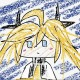in life

# Artificial Intelligence

## Resolution

If $l_i \Leftrightarrow \neg m_j$, then:

$\frac{l_1 \vee \cdots l_k \ \ \ \ m_1 \vee \cdots \vee m_n}{l_1 \vee \cdots \vee l_{i-1} \vee l_{i+1} \cdots \vee l_k \vee m_1 \vee \cdots \vee m_{j-1} \vee m_{j+1} \cdots \vee m_n}$

If there is a empty clause after a resolution, it means there are conflicts.

$$\frac{p\ \ \ \neg p}{\square}$$

Resolution algorithm to prove $\alpha$ within KB:

1. Generate CNF of the knowledge base $KB\wedge\neg\alpha$
• CNF: $(l_{1,1}\vee l_{1,2} \vee \cdots \vee l_{1,n_1}) \wedge (l_{2,1}\vee \cdots \vee l_{2,n_2})\vee\cdots \vee (l_{m,1}\vee\cdots\vee l_{m,n_m})$
• Also called k-CNF if $n_1 = n_2 = \cdots = n_m = k$
2. Repeat resolution process for every new rule
1. If there is no new rule, $\alpha$ cannot be proved.
2. If there is an empty literal, then stop and $\alpha$ is proved

Complexity: exponential

Expressiveness: better (not restricted to Horn clauses)

## Propositional logic

### Jargons

Use functions, predicates to represent relationships:

• A is a person -> $Person(A)$, Person: predicate
• Person a has a crippled left leg -> $Crippled(LeftLegOf(a))$, LeftLegOf: function (it’s OK since a human has only one left leg) Formally,

Term ::= Function ( Term {, Term}* ) AtomicSentence ::= predicate ( Term {, Term}* ) …

Forgot that.

# Networking

Cisco’s proprietary software, packettracer, couldn’t be downloaded without enrolling in its network course.

## IOS

• use enable to enable the thing
• show interfaces to have a look at all interfaces, show interface [interface].
• show vlan to show vlan
• configure terminal to open the configuration in terminal
• (config) interface [interface] to select an interface

## Dynamic Trunking Protocol

A proprietary protocol invented by Cisco to negotiate trunking on a link between two VLAN-aware switches.

There is several modes for it:

• access: puts the ethernet port into non-trunking mode and negotiates to convert the link to a nontrunk one, disregarding the neigboring port
• trunk: puts the ethernet port into trunking mode and negotiates to convert the link to a trunk one, disregarding the neigboring port
• dynamic auto: willing to trunk, will be trunk if the neighboring port is dynamic desirable or trunk
• dynamic desirable: actively willing to trunk
• nonegotiate: disable DTP. No wonder, it is not compatible with dynamic modes.

Except nonegotiate, all modes are triggered by (config-if) switchport mode [mode]. Switch to no-negotiate mode using (config-if) switchport nonegotiate.

Use a no before the statement to do the opposite thing, like:

(config-if) no shutdown: set up the link (config-if) no switchport nonegotiate: turn on DTP

# Computer Graphics

Blurring is often used in computer graphics, like, to create HDR(high dynamic range) bloom, depth of field or other post process effects.

HDR bloom: since the real-world camera cannot focus perfectly, so it will convolve the incoming image with an Airy disk. What about the Airy disk? Know what a spot of light looks like in our eyes? That would be one. A perfect lens with a circular aperture would make it because the diffraction of light.

This effect is not noticeable when it is not so bright. However, with more light we can see the blurred edge of the bright part of the image, taken by a camera. And computer graphics tries to reproduce the same effect by blurring.

You can think up an approach instantly: take the average of neighboring blocks, like below:

$$\begin{bmatrix} 1/9&1/9&1/9 \\ 1/9&1/9&1/9 \\ 1/9&1/9&1/9 \\ \end{bmatrix}$$ By all means this is bad. It will seem block-ish.

A better one is Gaussian blur. It uses the Gaussian distribution as the weight of neighboring pixels to calculate the average, or more accurately, do the convolution. The weight matrix, like the one above, is called kernel.

A 3x3 Gaussian kernel is like:

$$\begin{bmatrix} 1/16 & 1/8 & 1/16 \\ 1/8 & 1/4 & 1/8 \\ 1/16 & 1/8 & 1/16 \\ \end{bmatrix}$$

Some other kernels are here.

It also can be used before downsampling: Gaussian blur gets rid of the sharp edges in the image, thus after downsampling, it will free the image from aliasing like moire patterns. Aliasing happens because of the poor sampling makes different signals indistinguishable, and some high frequency signals might get in the way after sampling. So a low pass filter need to be applied before sampling.

Task: sample => downscale image Reason of problem: high freq. signal => ? Problem: aliasing => moire pattern Solution: low pass filter => blurring

And yes, Gaussian blur is also a low pass filter, with a Bode plot like a parabola. Possibly it seems obvious at first sight: Gaussian distribution has a factor $e^{-\frac{x^2+y^2}{2\sigma^2}}$, which is stable through Fourier transforms.

We can calculate it out. Let’s put the basic calculus into practice. \begin{aligned} &\iint e^{i\omega x}\cdot\frac{e^{-\frac{x^2+y^2}{2\sigma^2}}}{2\pi\sigma^2}\ dxdy\ =&e^{-2\sigma^2\omega^2}\iint \frac{e^{-\frac{(x-2\sigma^2\omega i)^2+y^2}{2\sigma^2}}}{2\pi\sigma^2}\ dxdy\ =&e^{-2\sigma^2\omega^2} \end{aligned} So the amplification is: \begin{aligned} dB&=20\log{e^{-2\sigma^2\omega^2}}\ &=-\frac{40}{\ln 10}\cdot \sigma^2\omega^2 \end{aligned} Perfect! It is a parabola after all.

Leave Kawase to the next day.

# Misc

マシンガンでも壊せなくて キャタピラーでも潰せなくて

# 1984

Ignorance is strength.

Big Brother包揽一切。

• page 1 of 1#### NoirGif

A progamer.

Student(probably)